# NEET Questions Solved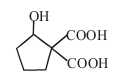$\underset{\mathrm{ii}·∆}{\overset{\mathrm{i}·{\mathrm{K}}_{2}{\mathrm{Cr}}_{2}{\mathrm{O}}_{7}}{\to }}$X; X is

(1)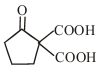(2)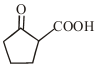(3)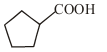(4)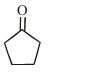$\mathrm{\beta }$-keto acid shows decarboxylation$\stackrel{{\mathrm{K}}_{2}{\mathrm{Cr}}_{2}{\mathrm{O}}_{7}}{\to }$$\stackrel{∆}{\to }$$\stackrel{∆}{\to }$Difficulty Level:

• 25%
• 37%
• 13%
• 27%